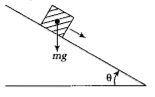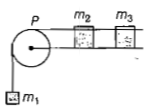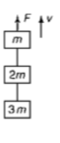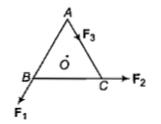A block A of mass m1 rests on a horizontal table. A light string connected to it passes over a frictionless pulley at the edge of table and from its other end another block B of mass m2 is suspended. The coefficient of kinetic friction between the block and the table is  μk. When the block A is sliding on the table, the tension in the string is

1. (m2km1)g /(m1+m2)

2. (m2km1)g/(m1+m2)

3. m1m2(1+μk)g/(m1+m2)

4. m1m2(1-μk)g/(m1+m2)

Concept Questions :-

Friction
High Yielding Test Series + Question Bank - NEET 2020

Difficulty Level:

A plank with a box on it at one end is gradually raised about the other end. As the angle of inclination with the horizontal reaches 30°, the box starts to slip and slides 4.0 m down the plank in 4.0 s. The coefficients of static and kinetic friction between the box and the plank will be. respectively1. 0.6 and 0.6

2. 0.6 and 0.5

3. 0.5 and 0.6

4. 0.4 and 0.3

Concept Questions :-

Friction
High Yielding Test Series + Question Bank - NEET 2020

Difficulty Level:

Two stones of masses m and 2m are whirled in horizontal circles, the heavier one in a radius r/2 and the lighter one in radius r. The tangential speed of lighter stone is n times that of the value of heavier stone when they experience same centripetal forces. The value of n is

1. 2

2. 3

3. 4

4. 1

Concept Questions :-

Uniform circular motion
High Yielding Test Series + Question Bank - NEET 2020

Difficulty Level:

A system consists of three masses m1,m2 and m3 connected by a string passing over a pulley P. The mass ${m}_{1}$ hangs freely and m2 and m3 are on a rough horizontal table (the coefficient of friction=μ) The pulley is frictionless and of negligible mass. The downward acceleration of mass m1, is (Assume,m1=m2=m3=m)1. g(1-gμ)/9

2. 2gμ/3

3. g(1-2μ)/3

4. g(1-2μ)/2

Concept Questions :-

Friction
High Yielding Test Series + Question Bank - NEET 2020

Difficulty Level:

A balloon with mass m is descending down with an acceleration a (where a < g). How much mass should be removed from it so that it starts moving up with an acceleration a?

1. 2ma/g+a

2. 2ma/g-a

3. ma/g+a

4. ma/g-a

Concept Questions :-

Application of laws
High Yielding Test Series + Question Bank - NEET 2020

Difficulty Level:

Three blocks with masses m,2m and 3m are connected by strings, as shown in the figure. After an upward force F is applied on block m, the masses move upward at constant speed v. What is the net force on the block of mass 2m? (g is the acceleration due to gravity)1. Zero

2. 2mg

3. 3mg

4. 6mg

Concept Questions :-

Types of forces
High Yielding Test Series + Question Bank - NEET 2020

Difficulty Level:

The upper half of an inclined plane of inclination θ is perfectly smooth while the lower half is rough. A block starting from rest at the top of the plane will again come to rest at the bottom if the coefficient of friction between the block and lower half of the plane is given by

1. μ=1/tanθ

2. μ=2/tanθ

3. μ=2tanθ

4. μ=tanθ

Concept Questions :-

Friction
High Yielding Test Series + Question Bank - NEET 2020

Difficulty Level:

A car of mass 1000 kg negotiates a banked curve of radius 90m on a frictionless road. If the banking angle is $45°$,the speed of the car is

1. 20 $m{s}^{-1}$                                             2. 30 $m{s}^{-1}$

3. 5 $m{s}^{-1}$                                               4. 10 $m{s}^{-1}$

Concept Questions :-

Friction
High Yielding Test Series + Question Bank - NEET 2020

Difficulty Level:

ABC is an equilateral triangle with O as its centre.  represent hree forces acting along the sides AB, BC and AC respectively. If the total torque about O is zero then the magnitude of ${F}_{3}$ is(a)${F}_{1}+{F}_{2}$                                   (b)${F}_{1}-{F}_{2}$

(c)$\frac{{F}_{1}+{F}_{2}}{2}$                                 (d)$2\left({F}_{1}+{F}_{2}\right)$

Concept Questions :-

Application of laws
High Yielding Test Series + Question Bank - NEET 2020

Difficulty Level:

A stone is dropped from a height h.

It hits the ground with a certain momentum

p. If the same stone is dropped from a height

100% more than the previous height, the

momentum when it hits the ground will

change by

(a) 68%                   (b) 41%

(c) 200%                 (d) 100%

Concept Questions :-

Newton laws